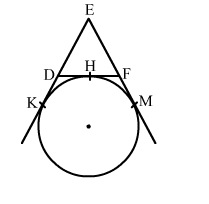# In the given figure, a circle touches the side DF of △EDF at H and touches ED and EF produced at K and M respectively.`
Question:

In the given figure, a circle touches the side DF of △EDF at H and touches ED and EF produced at K and M respectively. If EK = 9 cm then the perimeter of △EDF is
(a) 9 cm
(b) 12 cm
(c) 13.5 cm
(d) 18 cmSolution:

We know that tangent segments to a circle from the same external point are congruent.
Therefore, we have
EK = EM = 9 cm
Now, EK + EM = 18 cm
⇒ ED + DK + EF + FM = 18 cm
⇒ ED + DH + EF + HF = 18 cm           (∵DK = DH and FM = FH)
⇒ ED + DF + EF = 18 cm
⇒ Perimeter of △EDF = 18 cm
Hence, the correct answer is option (d)